Courses

# Types of Vectors and Scalars - Motion in a Plane, Class 11, Physics | EduRev Notes

## Class 11 : Types of Vectors and Scalars - Motion in a Plane, Class 11, Physics | EduRev Notes

The document Types of Vectors and Scalars - Motion in a Plane, Class 11, Physics | EduRev Notes is a part of the Class 11 Course Physics Class 11.
All you need of Class 11 at this link: Class 11

1. Scalar

In physics we deal with two types of physical quantities one is scalar and other is vector. Each scalar quantity has a magnitude and a unit.

For example Mass = 4 kg

Magnitude of mass = 4

and unit of mass = kg

Example of scalar quantities: Mass, Speed, Distance etc.

Scalar quantities can be added, subtracted and multiplied by simple laws of algebra.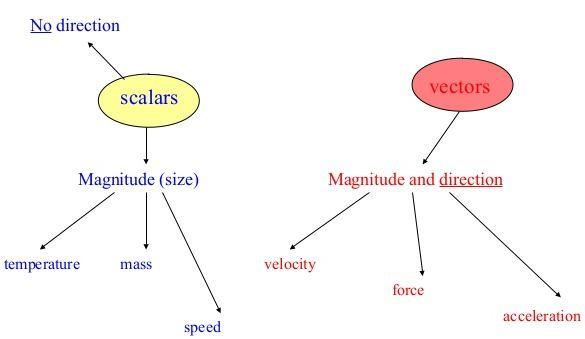Fig: Scalars and vectors

2. Vector

Vector are the physical quantities having magnitude as well as specified direction.

For example:

Speed = 4 m/s (is a scalar)

Velocity = 4 m/s toward north (is a vector)

If someone wants to reach some location then it is not sufficient to provide information about the distance of that location it is also essential to tell him about the proper direction from the initial location to the destination.

The magnitude of a vector is the absolute value of a vector and is indicated by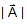or A.

Example of vector quantity : Displacement, velocity, acceleration, force etc.

Knowledge of direction: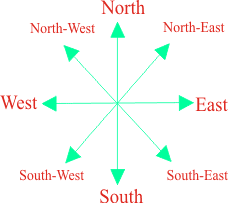Fig: Specified Direction

3. General Points Regarding Vectors

3.1 Representation of vector:

Geometrically, the vector is represented by a line with an arrow indicating the direction of vector as: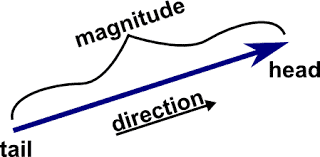Fig: Vector

Mathematically, vector is represented by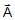.

Sometimes it is represented by bold letter A.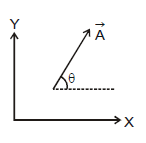Thus, the arrow in the above figure represents a vector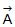in xy-plane making an angle θ with x-axis.

A representation of vector will be complete if it gives us direction and magnitude.

Symbolic form: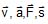used to separate a vector quantity from scalar quantities (u, i, m)

Graphical form: A vector is represented by a directed straight line,having the magnitude and direction of the quantity represented by it.

Example: If we want to represent a force of 5 N acting 45° N of E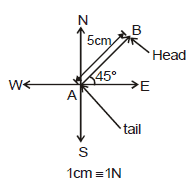(i) We choose direction coordinates.

(ii) We choose a convenient scale like 1 cm ≡ 1 N

(iii) We draw a line of length equal in magnitude and in the direction of vector to the chosen quantity.

(iv) We put arrow in the direction of vector.

Magnitude of vector: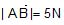3.2 Angle between two Vectors (θ):

Angle between two vectors means smaller of the two angles between the vectors when they are placed tail to tail by displacing either of the vectors parallel to itself (i.e 0 £ q £ p).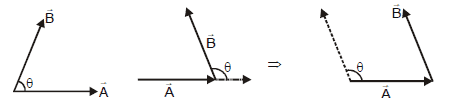Ex.1 Three vectors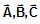are shown in the figure. Find angle between (i)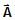and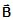, (ii)and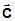, (iii)and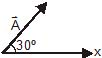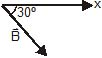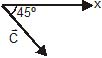Ans: To find the angle between two vectors we connect the tails of the two vectors. We can shift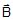&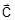such that tails of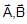and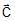are connected as shown in figure.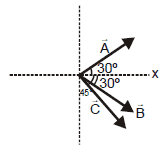Now we can easily observe that angle betweenandis 60º,andis 15º and betweenandis 75º.

3.3 Negative of Vector:

It implies vector of same magnitude but opposite in direction.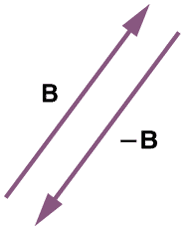3.4 Equality of Vectors:

Vectors having equal magnitude and same direction are called equal vectors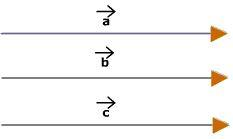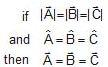3.5 Collinear vectors:

Any two vectors are collinear then one can be expressed in the terms of others.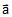=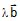(where λ is a constant)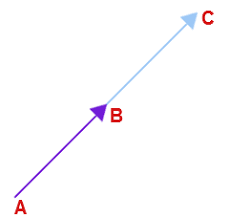3.6 Co-initial vector: If two or more vector start from the same point then they called co-initial vectors.
Example: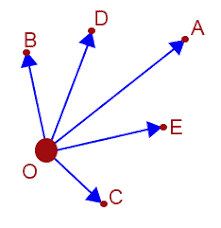Here A, B, C, D are co-initial vectors.

3.7 Coplanar vectors:

Three (or more) vectors are called coplanar vectors if they lie in the same plane or are parallel to the same plane. Two (free) vectors are always coplanar.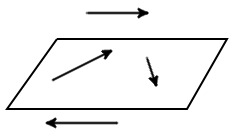Important points:

• If the frame of reference is translated or rotated the vector does not change (though its components may change).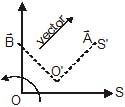3.8 Multiplication and division of a vector by a scalar:

Multiplying a vectorwith a positive number λ gives a vector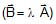whose magnitude become λ times but the direction is the same as that of.
Multiplying a vectorby a negative number λ gives a vectorwhose direction is opposite to the direction ofand whose magnitude is λ times.

The division of vectorby a non-zero scalar 'm' is defined as multiplication ofby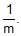At hereandare colinear vector

Ex.2 A physical quantity (m = 3 kg) is multiplied by a vector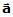such that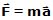. Find the magnitude and direction of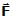if

(i)= 3m/s2 East wards

(ii)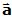= -4 m/s2 North wards

Ans:
(i)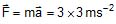East wards

= 9 N East wards

(ii)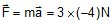North wards

= -12 N North wards

= 12 N South wards

Offer running on EduRev: Apply code STAYHOME200 to get INR 200 off on our premium plan EduRev Infinity!

,

,

,

,

,

,

,

,

,

,

,

,

,

,

,

,

,

,

,

,

,

,

,

,

,

,

,

;